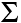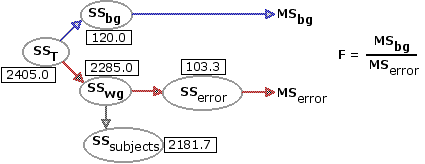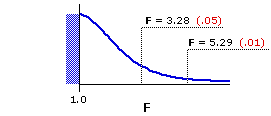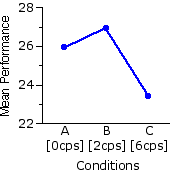Chapter 15.
One-Way Analysis of Variance for Correlated Samples
Part 2

• From the example introduced in Chapter 15, Part 1:

 A[0cps] B[2cps] C[6cps] All groupscombined Na=18 -∑Xai=466 -∑X2ai=12800Ma=25.9SSa=735.8 Nb=18 -∑Xbi=485 -∑X2bi=14021Mb=26.9SSb=952.9 Nc=18 -∑Xci=421 -∑X2ci=10443Mc=23.4SSc=596.3 NT=54 -∑XTi=1372 -∑X2Ti=37264MT=25.4SST=2405.0 SST = 2405.0 SSwg =TSSa+SSb+SSc = 735.8+952.9+596.3 = 2285.0 SSbg =TSST—SSwg = 2405.0—2285.0 = 120.0

¶Identification and Removal of SSsubj

The first five columns of the following table are a somewhat streamlined version of the data table you saw in Part 1. The means for the individual subjects are now highlighted in blue, and next to each subject's mean, in a new column, is a calculation whose general form you will surely recognize from Chapter 14. The difference between each subject's mean and the mean of the total array of data is taken as a deviate:
 deviate = Msubj* — MT We will use the subscript "subj*" to mean "any particular subject." The first subject would be subj1; the second, subj2; and so on.
The square of that difference is accordingly a squared deviate:
 squared deviate = (Msubj* — MT)2
And that squared deviate is then weighted by k=3, which is the number of measures (Xai, Xbi, and Xci) on which the subject's mean is based.
 weighted squared deviate = k(Msubj* — MT)2
It is the same general structure we used in Chapter 14 to calculate the value of SSbg from scratch, to measure the aggregate differences among the k groups. Now what we come out with is SSsubj, which is a measure of the aggregate differences among the 18 individual subjects.

 subjects A B C SubjectMeans 212223242526272829210211212213214215216217218 235232233232 231229229227 227228227227 224224217217 214213 239235232232 233230231229 231227227226 229225216215 215213 232231228229 226229227227 224224223223 219219218217 212213 235.3 232.7 231.0 231.0  230.0 229.3 229.0 227.7  227.3 226.3 225.7 225.3  224.0 222.7 217.0 216.3  213.7 213.0 3(35.3—25.4)2=294 3(32.7—25.4)2=159.9 3(31.0—25.4)2=94.1 3(31.0—25.4)2=94.1 3(30.0—25.4)2=63.5 3(29.3—25.4)2=45.6 3(29.0—25.4)2=38.9 3(27.7—25.4)2=15.9 3(27.3—25.4)2=10.8 3(26.3—25.4)2=2.4 3(25.7—25.4)2=0.3 3(25.3—25.4)2=0 3(24.0—25.4)2=5.9 3(22.7—25.4)2=21.9 3(17.0—25.4)2=211.7 3(16.3—25.4)2=248.4 3(13.7—25.4)2=410.7 3(13.0—25.4)2=461.3 MT=25.4 SSsubj=2179.4

I show you this particular method for calculating SSsubj only because it gives a clear idea of the conceptual structure of the measure. It would not be the method to use for practical computational purposes, as it is likely to end up with a considerable accumulation of rounding error. Also, if you are doing it by hand, it can be rather laborious. The following method is somewhat less laborious and will in any event minimize the risk of rounding errors. You will recognize it as analogous to the computational formula for SSbg that we worked through in Part 1; namely

 SSbg = (∑Xai)2Na + (∑Xbi)2Nb + (∑Xci)2Nc — (∑XTi)2NT

Except now the string of items to the left of the minus sign is replaced by another string that looks like this:

 (∑Xsubj1)2k + (∑Xsubj2)2k + (∑Xsubj3)2k + . . . and so on, for as many subjects as you have in the analysis.

That is: For each subject in the analysis, take the sum of that subject's scores across all k conditions, square that sum, and then divide the result by k, which is the number of scores on which the sum is based.

Since k is the same for each subject, this portion of the formula to the left of the minus sign can be rewritten as(∑Xsubj*)2k That is: For each subject in the analysis, take the sum of that subject's scores across all k conditions, square that sum, add up these squared sums across all subjects, and then divide the total of squared sums by k.

and the entire formula can then be written more compactly as

 SSsubj =(∑Xsubj*)2k — (∑XTi)2NT

The next version of our data table shows the sum for each subject, along with the calculation of the square of each subject's sum.

 subjects A B C SubjectSums (∑Xsubj*)2 212223242526272829210211212213214215216217218 235232233232 231229229227 227228227227 224224217217 214213 239235232232 233230231229 231227227226 229225216215 215213 232231228229 226229227227 224224223223 219219218217 212213 2106 298 293 293  290 288 287 283  282 279 277 276  272 268 251 249  241 239 1062=11236 982=9604 932=8649 932=8649 902=8100 882=7744 872=7569 832=6889 822=6724 792=6241 772=5929 762=5776 722=5184 682=4624 512=2601 492=2401 412=1681 392=1521(∑Xsubj*)2=111122

Recalling that XTi=1372 and NT=54, our calculation of SSsubj is accordingly

 SSsubj =(∑Xsubj*)2k — (∑XTi)2NT = 1111223 — (1372)254 = 2181.7

As always, one must be careful not to get so lost in the calculations as to lose sight of the concepts. What we have just calculated with SSsubj is the amount of variability within the total array of data that derives from individual differences among the subjects. And now that this amount has been identified, it can be removed. The process of removal is one of simple subtraction. The total amount of within-groups variability is SSwg=2285.0. Of this amount, SSsubj=2181.7 is attributal not to sheer, cussed random variability, but to extraneous pre-existing individual differences among the 18 subjects. Remove the latter from the former, and what remains is

 SSerror = SSwg—SSsubj = 2285.0—2181.7 = 103.3

Here is one of the same diagrams you saw in Part 1, except now the specific numerical values have been included for the several values of SS. The bottom line is that a huge chunk of SSwg is attributable to individual differences among the subjects, and that chunk is now removed. From this point on, it is all a smooth ride across familiar terrain.¶Calculation of MSbg, MSerror, and F

We begin by sorting out the several components of degrees of freedom. The first three are the same as we would have with a one-way independent-samples ANOVA:
 dfT = NT—1 = 54—1 = 53 Note that:T dfT = dfbg+dfwg dfbg = k—1 = 3—1 = 2 and dfwg = NT—k = 54—3 = 51
The only difference is that now we break dfwg into two further components, corresponding respectively to the two components of within-groups variability, SSsubj and SSerror. For dfsubj the basic concept is once again "the number of items minus one." In this case the number of items is the number of subjects, which we will designate as Nsubj. Thus
 dfsubj = Nsubj—1 = 18—1 = 17
Remove this component from dfwg, and what remains is
 dferror = dfwg—dfsubj = 51—17 = 34

With these, we can then calculate the two relevant MS values as
 MSbg = SSbgdfbg = 120.02 = 60.0
and
 MSerror = SSerrordferror = 103.334 = 3.0
which in turn give us
 F = MSbgMSerror = 60.03.0 = 20.0 with df=2,34

Figure 15.2 shows the sampling distribution of F for df=2,34, and the adjacent table shows the corresponding portion of Appendix D. As indicated, F=3.28 and F=5.29 mark the points in this distribution beyond which fall 5% and 1%, respectively, of all possible mere-chance outcomes, assuming the null hypothesis to be true.

Figure 15.2. Sampling Distribution of F for df=2,34dfdenomi-nator df numerator 1 2 3 34 4.137.44 3.285.29 2.884.42

 As the observed value of F=20.0 falls far to the right of F=5.29, the aggregate differences among the means of the three groups of measures, A|B|C, can be adjudged significant well beyond the .01 level. Our investigator can accordingly reject the null hypothesis, concluding with a high degree of confidence that the aggregate mean differences among the three groups of measures stem from something more than mere chance coincidence.• ANOVA Summary Table
 Source SS df MS F P between groups  ("effect") 120.0 2 60.0 20.0 <.01 within groups 2285.0 51 ·error 103.3 34 3.0 ·subjects 2181.7 17 TOTAL 2405.0 53

The assumptions and step-by-step computational procedures for the one-way correlated-samples ANOVA will be outlined in Part 3 of this chapter. It is also in Part 3 that we will see how the Tukey HSD test can be adapted to perform pairwise comparisons among the individual group means in a correlated-samples ANOVA.

• End of Chapter 15, Part 2.